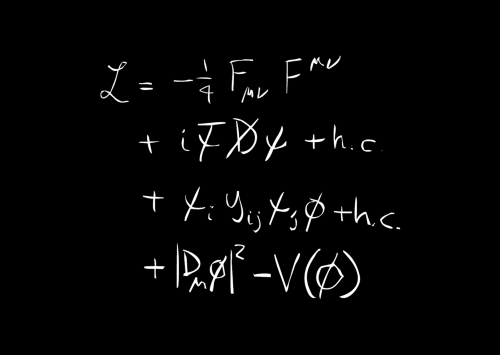# Standard Model formula postcardLagrangian of the Standard Model of particle physics

This formula is the mathematical representation of the Standard Model of particle physics, the theory that describes the elementary particles and the forces that bind them together. The first line describes the forces: electricity, magnetism, the strong nuclear force and the weak nuclear force. The second line shows how these forces act on the elementary particles of matter. The last two lines describe the Higgs boson and the mechanism by which particles acquire their mass.

Price
1 CHF
Topic
Standard Model Formula
Category
Postcards
Technical Specifications

Format : 15 x 10.5 cm